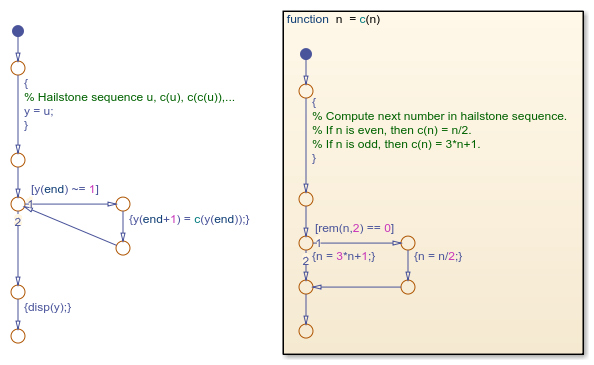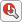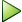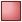## 将 MATLAB 代码转换为 Stateflow 流程图

• `if``if-else` 及其他嵌套决策语句。

• `for``while` 循环。

• `switch` 语句。

• MATLAB 函数转换为 Stateflow 图形函数。

• MATLAB 脚本转换为 Stateflow 流程图。

### 根据 MATLAB 脚本创建流程图

• 如果 ni 为偶数，则 ni+1 = ni/2

• 如果 ni 为奇数，则 ni+1 = 3ni + 1

Collatz 猜想指出，每个正整数都有一个最终达到 1 的冰雹序列。

```% Hailstone sequence u, c(u), c(c(u)),... y = u; while y(end) ~= 1 y(end+1) = c(y(end)); end disp(y); function n = c(n) % Compute next number in hailstone sequence. % If n is even, then c(n) = n/2. % If n is odd, then c(n) = 3*n+1. if rem(n,2) == 0 n = n/2; else n = 3*n+1; end end```

1. 打开一个新的独立图。

`edit hailstone.sfx`
2. 状态图选项卡上，选择 >

3. 在对话框中，选择 MATLAB 脚本，然后点击打开。构型向导会在您的 Stateflow 图中添加一个流程图和一个图形函数。双击图形函数以查看其内容。4. 符号窗格中，点击。Stateflow 编辑器将 `u``y` 解析为局部数据。

5. 保存您的图。

6. 要从 Stateflow 编辑器中执行图，请在符号窗格中输入值 `u` = 9，然后点击。当流程图正在执行时，Stateflow 编辑器通过图动画突出显示激活的转移。当执行停止时，MATLAB 命令行窗口会显示从值 9 开始的冰雹序列：

``` 9 28 14 7 22 11 34 17 52 26 13 40 20 10 5 16 8 4 2 1 ```
7. 点击